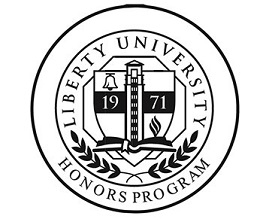## Senior Honors Theses

Spring 5-3-2018

#### School

College of Arts and Sciences

Mathematics

#### Keywords

Hyperbolic, Fourier, Associative Real Algebra, Wave Equation

#### Disciplines

Other Applied Mathematics | Other Mathematics

#### Abstract

The complex numbers have proven themselves immensely useful in physics, mathematics, and engineering. One useful tool of the complex numbers is the method of conformal mapping which is used to solve various problems in physics and engineering that involved Laplace’s equation. Following the work done by Dr. James Cook, the complex numbers are replaced with associative real algebras. This paper focuses on another algebra, the hyperbolic numbers. A solution method like conformal mapping is developed with solutions to the one-dimensional wave equation. Applications of this solution method revolve around engineering and physics problems involving the propagation of waves. To conclude, a series of examples and transformations are given to demonstrate the solution method.

COinS